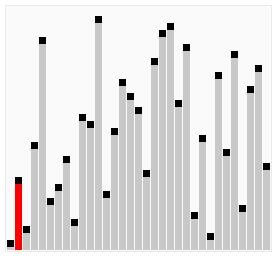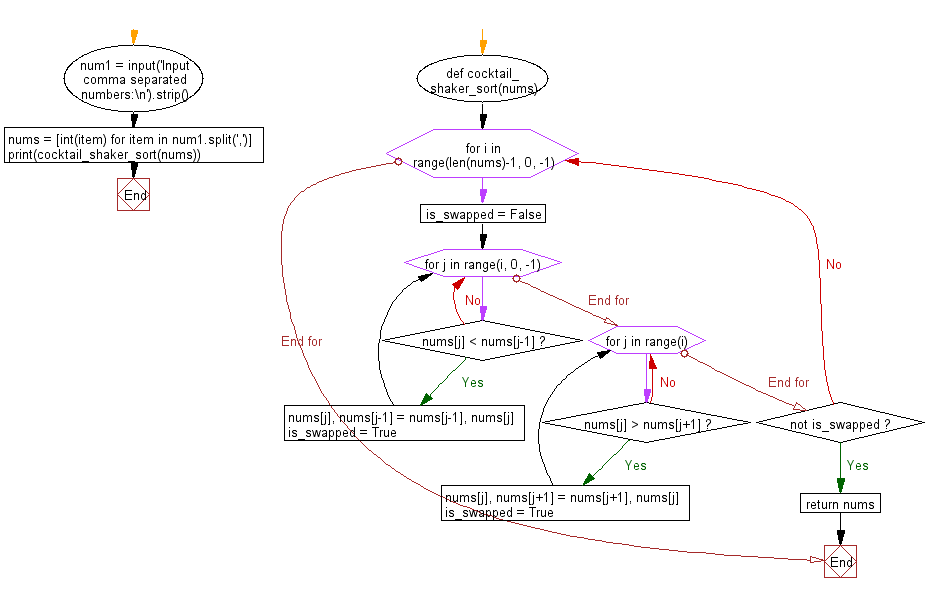﻿ Python: Sort a list of elements using Cocktail shaker sort - w3resource# Python: Sort a list of elements using Cocktail shaker sort

## Python Search and Sorting : Exercise-14 with Solution

Write a Python program to sort a list of elements using Cocktail shaker sort.
From Wikipedia, Cocktail shaker sort, also known as bidirectional bubble sort, cocktail sort, shaker sort (which can also refer to a variant of selection sort), ripple sort, shuffle sort, or shuttle sort, is a variation of bubble sort that is both a stable sorting algorithm and a comparison sort. The algorithm differs from a bubble sort in that it sorts in both directions on each pass through the list. This sorting algorithm is only marginally more difficult to implement than a bubble sort, and solves the problem of turtles in bubble sorts. It provides only marginal performance improvements, and does not improve asymptotic performance; like the bubble sort, it is not of practical interest (insertion sort is preferred for simple sorts), though it finds some use in education.

Visualization of shaker sort:Sample Solution:

Python Code:

``````def cocktail_shaker_sort(nums):
for i in range(len(nums)-1, 0, -1):
is_swapped = False

for j in range(i, 0, -1):
if nums[j] < nums[j-1]:
nums[j], nums[j-1] = nums[j-1], nums[j]
is_swapped = True

for j in range(i):
if nums[j] > nums[j+1]:
nums[j], nums[j+1] = nums[j+1], nums[j]
is_swapped = True

if not is_swapped:
return nums

num1 = input('Input comma separated numbers:\n').strip()
nums = [int(item) for item in num1.split(',')]
print(cocktail_shaker_sort(nums))
```
```

Sample Output:

```Input comma separated numbers:
15, 37, 69, 26, 78
[15, 26, 37, 69, 78]
```

Flowchart:Python Code Editor:

What is the difficulty level of this exercise?

Test your Programming skills with w3resource's quiz.

﻿

## Python: Tips of the Day

Use Reversed() In for Loops:

```>>> tasks = ['laundry', 'picking up kids', 'gardening', 'cooking']